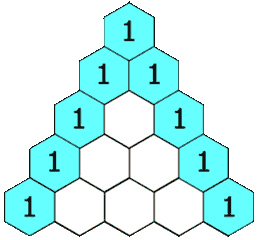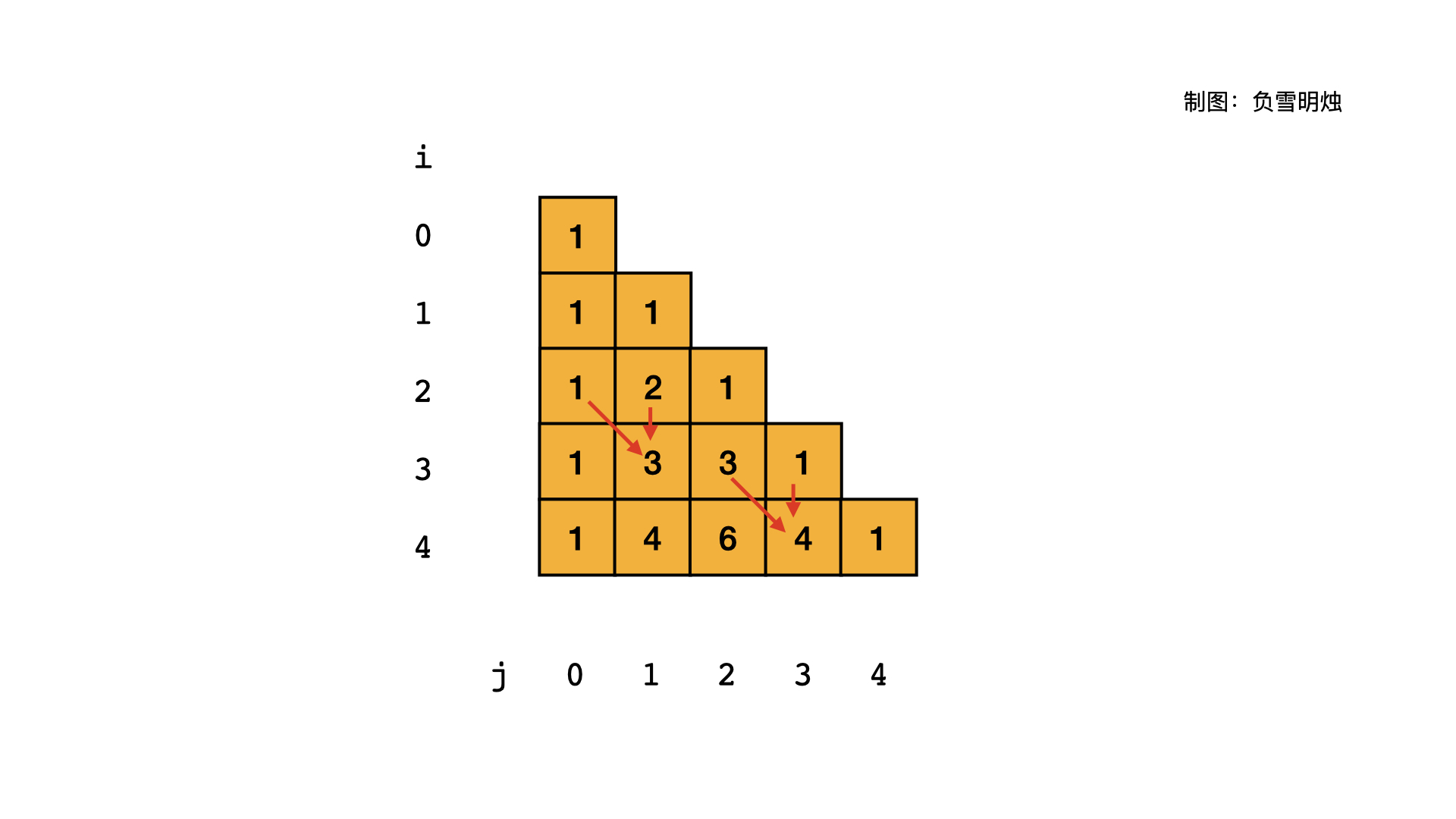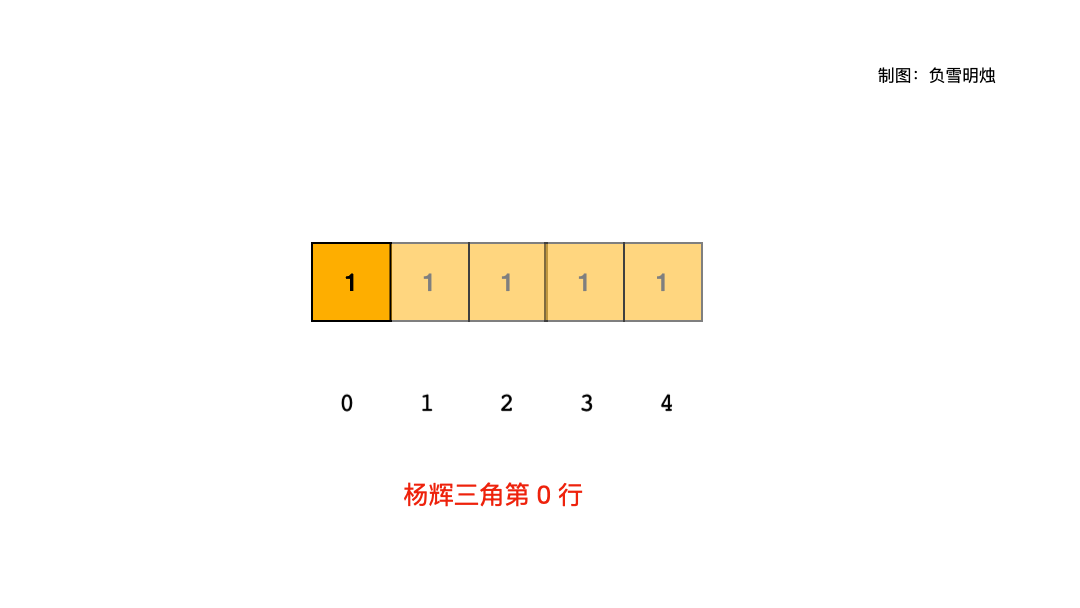# 119. Pascal's Triangle II 杨辉三角 II

@TOC

[LeetCode]

Total Accepted: 74643 Total Submissions: 230671 Difficulty: Easy

# # 题目描述

Given a non-negative index k where k ≤ 33, return the kth index row of the Pascal's triangle.

Note that the row index starts from 0.In Pascal's triangle, each number is the sum of the two numbers directly above it.

Example:

Input: 3
Output: [1,3,3,1]


Could you optimize your algorithm to use only O(k) extra space?

# # 解题思路

## # 方法一： 空间复杂度class Solution(object):
def getRow(self, rowIndex):
"""
:type rowIndex: int
:rtype: List[int]
"""
res = [[1 for j in range(i + 1)] for i in range(rowIndex + 1)]
for i in range(2, rowIndex + 1):
for j in range(1, i):
res[i][j] = res[i - 1][j - 1] + res[i - 1][j]
return res[-1]


## # 方法二：空间复杂度class Solution(object):
def getRow(self, rowIndex):
"""
:type rowIndex: int
:rtype: List[int]
"""
res =  * (rowIndex + 1)
for i in range(2, rowIndex + 1):
for j in range(i - 1, 0, -1):
res[j] += res[j - 1]
return res


# # 刷题心得

1. 本题的空间优化方式，类似于滚动数组，看来刷题的方法是通用的。
2. 本题也可以用公式求解。

# # 日期

2016 年 05月 8日 2018 年 11 月 21 日 —— 又是一个美好的开始 2021 年 2 月 12 日 —— 今天是大年初一，祝大家牛年大吉！

: https://leetcode.com/problems/pascals-triangle-ii1]: https://leetcode.com/problems/pascals-triangle/